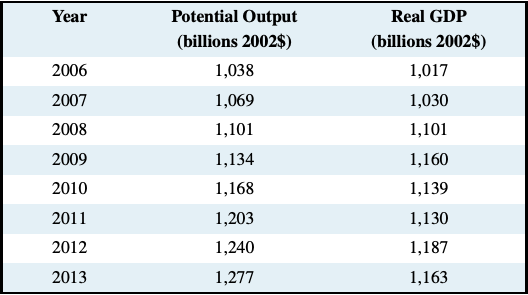# Exercises for Chapter 5

Exercise 5.1 Suppose we have the following information for an economy:(a) Plot the AD and AS curves in a carefully labeled diagram.

(b) What are the short-run equilibrium values of real GDP and the price level?

Exercise 5.2 Suppose we learn that potential output is 500 for the economy in Exercise 5.1.

(a) Add a line to your diagram for Exercise 5.1 to illustrate potential GDP.

(b) What is the size of any output gap you see in the diagram?

(c) What is the size and sign of the output gap?

Exercise 5.3 Potential GDP is determined by the size of the labour force, the stock of capital and the state of technology used in the production process. Assume the labour force grows over time, and research and development lead to improvements in technology, and productivity. Use an AD/AS diagram to illustrate potential GDP both before and after the growth in labour force and the improvement in technology.

Exercise 5.4 Consider an economy described by the following: AD: $$Y = 2250 − 10P$$, AS: $$P = 125 + 0.1Y$$.

(a) What are the short run equilibrium values for real GDP and the price level?

(b) Assume potential output is 500 and draw an AD/AS/YP diagram to show the initial short run equilibrium real GDP, price level and potential output.

(c) Changes in international market conditions drive up prices for crude oil and base metals. Increased production costs driven by these higher input prices raise the general price level by 5 at every level of output. Write the equation of the new AS curve. What are the new short run equilibrium real GDP and price level?

(d) Draw the new AS curve in your diagram for b). What is the size of the output gap?

Exercise 5.5 Growth in potential output is determined by growth in the labour force and growth in labour productivity. Suppose the labour force grows by 1.5 percent a year and labour productivity, based on increased capital and improved technology, grows by 1.0 percent a year.

(a) What is the annual growth in potential output?

(b) Illustrate the growth in potential output in an AD/AS diagram.

(c) Aggregate demand is not changed by the change in potential output. Indicate any output gap caused by the change in potential output.

Exercise 5.6 Suppose we have the following data for an economy:Calculate the output gap for each year in this economy. Plot the output gap in a time series diagram. Date the timing of the phases of any business cycles you see in your plot of the output gap.

Exercise 5.7 Suppose employment in the economy is proportional to output based on the output function Y = 100N, where N is employment in person years. If a recessionary gap reduced output (Y) by 1.0 percent what change in employment would result?